Class

# Algorithm

Algorithm Class 알고리즘

## Data Abstraction and Basic Data Structures

### Abstract Data Type(ADT)

Abstract Data Type

• Structures - data structure declarations
• Functions - operation definitions

An ADT is identified as a Class

• in languages such as C++ and Java

Designing algorithms and proving correctness of algorithms

• based on ADT operations and specifications

### Binary Tree ADT

• A binary tree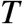is a set of elements, called nodes, that is empty or satisfies

1. There is a distinguished node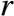called root
2. The remaining nodes are divided into two disjoint subsets,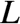and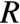, each of which is a binary tree(is called left subtree andis called the right subtree of)
• There are most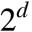nodes at depth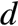of a binary tree

• A binary tree with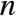nodes has height at least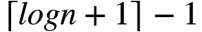• A binary tree with height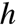has at most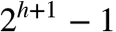nodes

### Stack

• A stack is a linear structure in which insertions and deletions are always made at one end, called top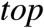• This updating policy is called last in, first out(LIFO)

### Queue

• A queue is alinear structure in which
• all insertions are done at one end, called the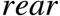or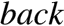• all deletions are done at the other end, called the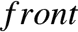• This updating policy is called first in, first out(FIFO)

### Priority Queue

• A priority queue is a structure with some aspects of FIFO queue but in which element order is related to each element’s priority, rather than its chronological arrival time
• The one element that can be inspected and removed is the most important element currently in the priority queue

### Union-Find ADT for Disjoint Sets

• Through a Union operations, two (disjoint) sets can be combined
• Let the set id of the original two set be,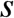and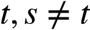• Then, new set has one unique set id that is eitheror $t • Through a Find operation, the current set id of an element can be retrieved • Often elements are integers and the set id is some particular element in the set, called the leader #### Operations • UnionFind create(int n) • create a set ofsingleton disjoint sets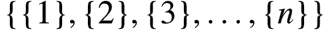• int find(unionFind sets, e) • return the set id for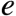• void makeSet(unionFind sets, int e) • union one singleton set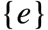(not already in the sets) • void union(unionFind sets, int s, int t) •and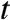are set ids,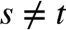• a new set is created by union of set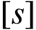and set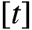• the new set id is eitheror, in some case min(s,t) ### Dictionary ADT • A dictionary is a general associative storage structure • Items in a dictionary • have an identifier • associated information that needs to be stored and retrieved • no order implied for identifiers in a dictionary ADT ## Sorting ### Insertion Sort Input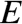, an array of elements, and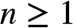, the number of elements. The range of indexes is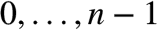Output, with elements in nondecreasing order of their keys void insertionSort(Element[] E, int n) int xindex; for(xindex=1; xindex<n; xindex++) Element current = E[xindex]; key x = current.key; int xLoc = shiftVacRec(E, xindex, x); E[xLoc] = current; return; ================================================ int shiftVacRec(Element[] E, int vacant, Key x) int xLoc; if(vacant == 0) xLoc = vacant; else if(E[vacant-1].key <= x) xLoc = vacant; else E[vacant] = E[vacant-1] xLoc = shiftVacRec(E, vacant-1, x); return xLoc; #### sourcecode ### Quick Sort Quick sort is a randomized sorting algorithm based on the divde-and-conquer paradigm • Divde Pick a random element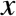(called pivot) and partition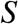into •elements less than•elements equal•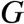elements greater than• Conquer sortand• Combine join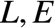and$G#### Partition

• Partition an input sequence as follows
• remove, in turn, each element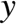fromand
• insertintoor, depending on the result of the comparison with the pivot• Each insertion and removal is at the beginning or at the end of a sequence, and hence takes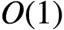time
• Thus, the partition step of quick-sort takes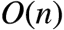time
Algorithm partition(S, p)
Input sequence S, position p of pivot
Output subsequences L, E, G of the elements of S less than, equal to, or greater than the pivot

L, E, G <- empty sequences
x <- S.remove(p)

while is not S.isEmpty()
y <- S.remove(S.first())
if y<x
L.insertLast(y)
else if y == x
E.insertLast(y)
else
G.insertLast(y)

return L, E, G

### Merge Sort

Problem
Given two sequences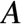and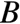sorted in nondecreasing order, merge them to create one sorted sequence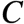Strategy
Determine the first item in C: It is the minimum between the first items ofand. Suppose it is the first item of. Then, the rest ofmust be the result of merging the rest ofwithMerge(A, B, C)
if(A is empty)
rest of C = rest of B
else if(B is empty)
rest of C = rest of A

else if(first of A <= first of B)
first of C = first of A
merge(rest of A, B, rest of C)

else
first of C = first of B
merge(A, rest of B, rest of C)
return

Input
Arrayand indexes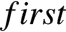, and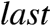, such that the elements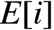are defined for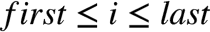Output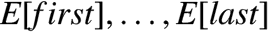is sorted rearrangement of the same elements

void mergeSort(Element[] E, int first, int last)

if(first<last)
int mid = (first+last)/2
mergeSort(E, first, mid);
mergeSort(E, mid+1, last)
merge(E, first, mid, last)

### Heap Sort

A Heap data structure is a binary tree with special properties

• Heap Structure
• Partial order tree property

#### Heap Structure

• A binary treeis a heap structure if an only if it satisfies the following conditions(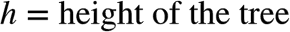)
1.is complete at least through depth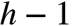2. All leaves are at depthor3. All paths to a leaf of depthare to the left of all paths to a leaf of depth• Such a tree is also called a left-complete binary tree

#### Partial order tree property

• A tree is a partial order tree if and only if the key at any node is greater than or equal to the keys at each of its children

#### Construct Heap

Input
A heap structure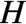that does not necessarily have the partial order tree property

Outputwith the same nodes rearranged to satisfy the partial order tree property

void constructHeap(H)

if(H is not leaf)
constructHeap(left subtree of H);
constructHeap(right subtree of H);
Element K = root(H);
fixHeap(H, K)

#### Sorting

• heapSort(E, n) // construct H from E, the set of n elements to be sorted
for(i=m; i>=1; i--)
curMax = getMax(H)
deleteMax(H)
E[i] = curMax;
• deleteMax(H)
• copy the rightmost element of the lowest level ofinto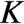• delete the rightmost element on the lowest level of• fixHeap(H,K)
• fixHeap(H, K)
if(H is leaf)
insert K in root(H);
else
set largerSubHeap to leftSubtree(H) or rightSubtree(H), whichever has larger key at its root. This involves one key comparison

if(K.key >= root(largerSubHeap.key))
insert K in root(H)
else
insert root(largerSubHeap) in root(H)
fixHeap(largerSubHeap, K)
returnProperties for example

• keys are names
• keys are all five-digit decimal integers
• keys are integers between 1 and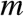For sorting each of these examples, the keys are

• distributed into different piles
• sort each pile individually
• combine all sorted piles

Algorithms that sort by such methods are not in the class of algorithms previously considered because

• to use them we must know something about either the structure or the range of the keys

#### Startegy

• If the keys are distributed into piles (also called buckets) first according to their least significant digits(or bits, letters, or fields)
• then the problem of sorting the buckets has been completely eliminated

#### Sorting

List radixSort(List, int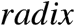, int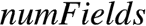)

List[] buckets = new List[radix];
int fields; // field number within the key
List newL;

for(field=0; field < numFields; field++)
Initialize buckets array to empty lists
distribute(newL, buckets, radix, field);
newL = combine(buckets, radix)
return newL;

void distribute(List, List[]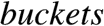, int, int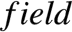)

//distribute keys into buckets

List remL;
remL = L;

while(remL is not null)
Element K = first(remL);
buckets[b] = cons(K, buckets[b]); //construct list
remL = rest(remL);
return

List combine(List[], int)

//Combine linked lists in all buckets into one list L

int b;
List L, remBucket;
L = null;
for(b = radix-1; b>=0; b--)
remBucket = buckets[b];
while(remBucket is not null)
key K = first(remBucket);
L = cons(K, L)
remBucket = rest(remBucket);
return L;#### 'Class' 카테고리의 다른 글

 [cs224n] Lecture 4 Word Window Classification and Neural Networks  (0) 2018.03.25 2018.03.10 2015.05.08 2015.05.08 2015.04.29 2015.04.18